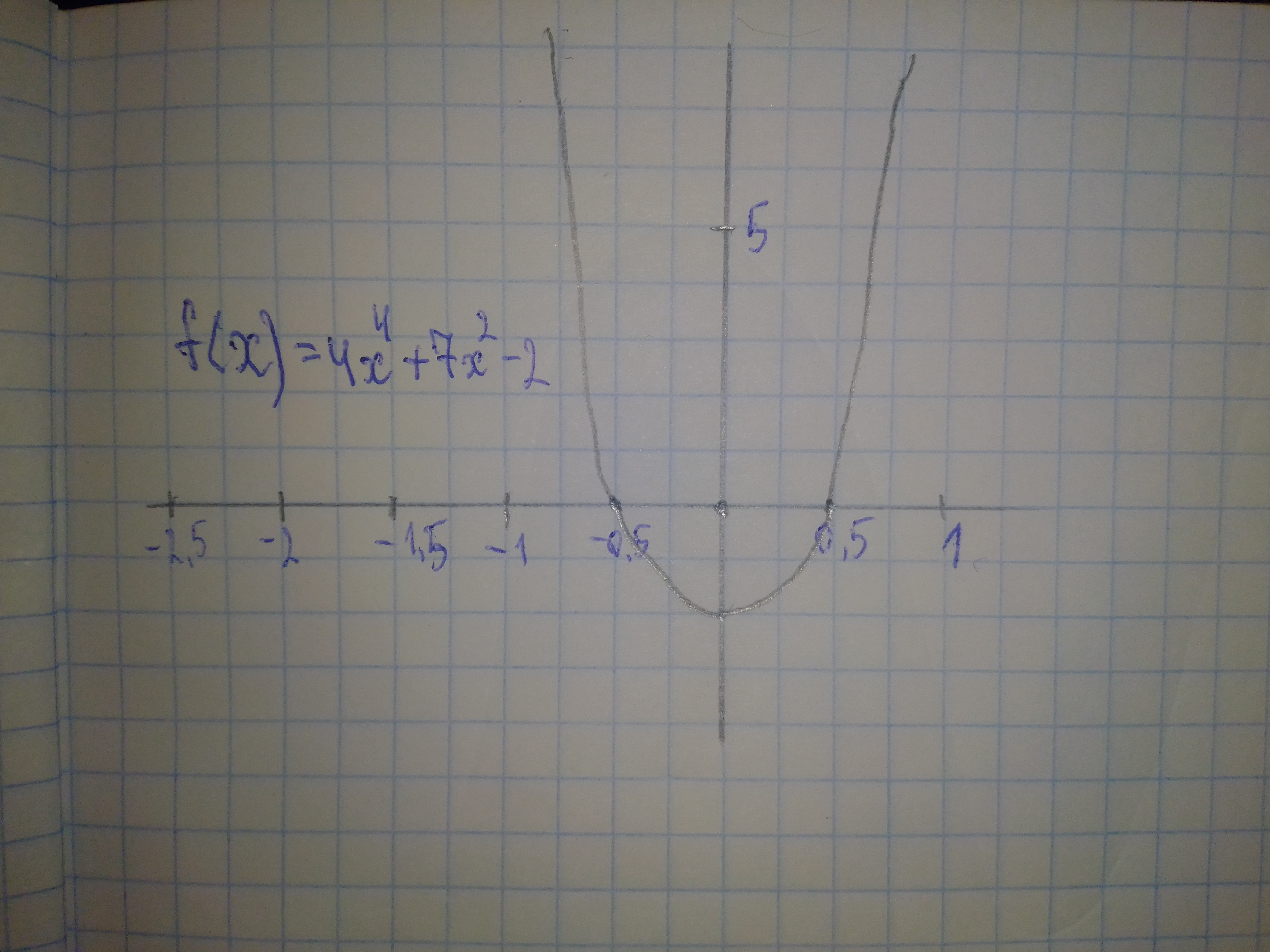Ask question

# Graph each polynomial function. f(x)=4x^{4}+7x^{2}-2# Graph each polynomial function. f(x)=4x^{4}+7x^{2}-2

Question
Polynomial graphsasked 2021-02-20
Graph each polynomial function. $$\displaystyle{f{{\left({x}\right)}}}={4}{x}^{{{4}}}+{7}{x}^{{{2}}}-{2}$$

## Answers (1)2021-02-21### Relevant Questionsasked 2020-10-18
Graph each polynomial function. PSKf(x)=x^{4}\ -\ x^{3}\ -\ 6x^{2}\ +\ 4x\ +\ 8 PSZasked 2021-02-26
a) Identify the parameters a, k, d, and c in the polynomial function $$\displaystyle{y}={\frac{{{1}}}{{{3}}}}{\left[-{2}{\left({x}+{3}\right)}\right]}^{{{4}}}-{1}$$. Describe how each parameter transforms the base function $$\displaystyle{y}={x}^{{{4}}}$$. b) State the domain and range, the vertex, and the equation of the axis of symmetry of the transformed function. c) Describe two possible orders in which the transformations can be applied to the graph of $$\displaystyle{y}={x}^{{{4}}}$$ to produce the graph of $$\displaystyle{y}={\frac{{{1}}}{{{3}}}}{\left[-{2}{\left({x}+{3}\right)}\right]}^{{{4}}}-{1}$$. d) Sketch graphs of the base function and the transformed function on the same set of axes.asked 2020-11-20
Graph each polynomial function. Factor first if the expression is not in factored form.
$$\displaystyle{f{{\left({x}\right)}}}={2}{x}^{{{3}}}{\left({x}^{{{2}}}−{4}\right)}{\left({x}−{1}\right)}$$asked 2020-12-03
Rational functions can have any polynomial in the numerator and denominator. Analyse the key features of each function and sketch its graph. Describe the common features of the graphs. $$\displaystyle{a}{)}{f{{\left({x}\right)}}}={\frac{{{x}}}{{{x}^{{{2}}}-{1}}}}\ {b}{)}{g{{\left({x}\right)}}}={\frac{{{x}-{2}}}{{{x}^{{{2}}}+{3}{x}+{2}}}}\ {c}{)}{h}{\left({x}\right)}={\frac{{{x}+{5}}}{{{x}^{{{2}}}-{x}-{12}}}}$$asked 2020-12-02
Graph each polynomial function.$$\displaystyle{f{{\left({x}\right)}}}={x}^{{{3}}}+{2}{x}^{{{2}}}-{5}{x}-{6}$$asked 2021-02-03
Graph each polynomial function. PSKf(x)=2x^{3}\ -\ x^{2}\ +\ 2x\ -\ 1 PSZasked 2020-11-23
Graph each polynomial function. $$\displaystyle{f{{\left({x}\right)}}}={x}^{{{4}}}-{4}{x}^{{{3}}}-{5}{x}^{{{2}}}+{14}{x}-{15}$$asked 2021-01-25
Using calculus, it can be shown that the secant function can be approximated by the polynomial $$\displaystyle{\sec{{x}}}\approx{1}+{\frac{{{x}^{{{2}}}}}{{{2}!}}}+{\frac{{{5}{x}^{{{4}}}}}{{{4}!}}}$$ where x is in radians. Use a graphing utility to graph the secant function and its polynomial approximation in the same viewing window. How do the graphs compare?asked 2021-01-10
Graph each polynomial function. Factor first if the expression is not in factored form. $$\displaystyle{f{{\left({x}\right)}}}={\left({4}{x}+{3}\right)}{\left({x}+{2}\right)}^{{{2}}}$$asked 2021-03-18
Sketch graphs of each of the following polynomial functions. Be sure to label the x- and they-intercepts of each graph. $$\displaystyle{a}.{y}={x}{\left({2}{x}+{3}\right)}{\left({2}{x}-{5}\right)}{b}.{y}={\left({11}-{2}{x}\right)}^{{{2}}}{\left({x}-{2}\right)}$$
...